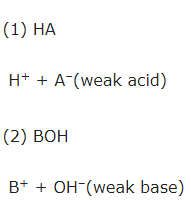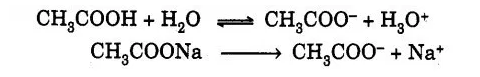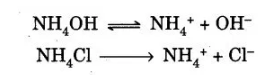Checkout JEE MAINS 2022 Question Paper Analysis : Checkout JEE MAINS 2022 Question Paper Analysis :

# Study The pH Change By Common-Ion In Case Of Weak Acids And Weak Bases

The Common ion effect is defined as the transfer in equilibrium that occurs due to the addition of an ion existing in the equilibrium reaction. As we know ionization for weak acid or a weak base is reversible and is represented as below.We can see an increase in the concentration of H+ ions in the first reaction. In the reverse direction, there is a shift in equilibrium in the second reaction. Considering the weak acid (acetic acid) and a weak base (ammonium hydroxide). When acetic acid is ionized by adding sodium acetate, the concentration of acetate ion increases. There is a shift in equilibrium in the reverse direction and the concentration of H3O+ ions decreases increasing the pH of the solution.In the case of ammonium hydroxide which is a weak base is partially dissociated. When ammonium chloride is added the concentration of NH4+ ions increases and is shown in the reaction below.

There is a shift in the equilibrium in the reverse direction and hence decrease in OH ion concentration is seen.## Aim:

To Learn the pH change by common-ion in case of weak acids and weak bases.

## Materials Required:

Test tube, test tube stand, pH paper, glass rod, acetic acid, sodium acetate, ammonium hydroxide, and ammonium chloride

## The procedure to determine the pH change on weak acid using a common ion:

1. Take 10 mL of acetic acid in a test tube and dip the pH paper and note the change in colour.
2. Compare the colour with the standard pH indicator chart to get the exact pH value.
3. Now add 1g of sodium acetate to the test tube.
4. Mix it well to dissolve sodium acetate in the acetic acid solution using a glass rod.
5. Determine the pH of the solution using pH paper.
6. Add 1g of sodium acetate to the test tube and find the pH of the solution.
7. Again add 1g of sodium acetate and find the change in the pH.

## Observation

pH value on weak acid after adding common ion

 SI.no sample Colour on pH paper Approximate pH value 1 CH3COOH 2 CH3COOH+1g CH3COONa 3 CH3COOH+2g CH3COONa 4 CH3COOH+3g CH3COONa

## The procedure to determine the pH change on the weak base using a common ion:

1. Take 10 mL of ammonium hydroxide solution in a test tube and dip the pH paper and note the change in colour.
2. Compare the colour with the standard pH indicator chart to get the exact pH value.
3. Now add 1.5g of ammonium chloride into the test tube.
4. Using a glass rod, mix the solution well to dissolve ammonium chloride in ammonium hydroxide solution.
5. Determine the pH of the solution using pH paper.
6. Add 1.5g of ammonium chloride to the test tube and find the pH of the solution again.
7. Again add 1.5g of ammonium chloride and find the change in the pH.

## Observation

pH value on weak base after adding common ion

 SI.no sample Colour on pH paper Approximate pH value 1 NH4OH 2 NH4OH+1.5g NH4Cl 3 NH4OH+3g NH4Cl 4 NH4OH+4.5g NH4Cl

## Precautions to be taken during the experiment:

• Match the colour of the pH paper exactly with the standard pH indicator chart.
• Note the increase and decrease in pH value in the experiment

## Viva Voice:

1. What is the ionic product of water

Kw = [H3O+][OH]

2. what happens to the pH of the solution if little acid is added to the water?

The concentration of H3O+ ions in the solution increases and hence the pH of the solution decreases.

3. Does the pH of the solution change upon adding a common ion to the weak acid solution

Yes, the pH of the solution increases.

4. What is the pH of sodium carbonate?

Greater than 7.

5. What does the pH of a solution indicate?

Amount of H3O+ ion concentration in moles per litre.

Test your knowledge on ph change by common ion in case of weak acids and weak bases experiment